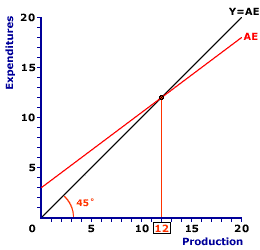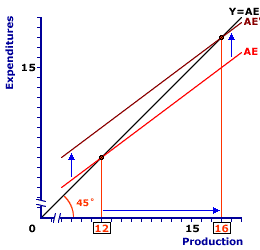Thursday  June 1, 2023
 AmosWEB means Economics with a Touch of Whimsy!PERSONAL INCOME: The total income received by the members of the domestic household sector, which may or may not be earned from productive activities during a given period of time, usually one year. The primary use of personal income is to measure the income actually paid out to the household sector. After adjusting for income taxes, personal income forms the basis for consumption expenditures on gross domestic product.MULTIPLIER, KEYNESIAN CROSS:

An analysis of the multiplier principle using the Keynesian cross intersection between the aggregate expenditures line and the 45-degree equilibrium guideline. The Keynesian cross analysis illustrates the Keynesian multiplier as a shift of the aggregate expenditures line and a subsequent change of the equilibrium level of aggregate production. This analysis illustrates the important role played by the slope of the aggregate expenditures line, and the thus the marginal propensity to consume. An alternate but comparable analysis of the multiplier principle is accomplished using the injections-leakages model.
The multiplier principle is the notion that relatively small changes in autonomous expenditures or other shocks cause relatively large overall changes in aggregate production and income. The Keynesian cross provides a handy framework for illustrating this multiplier principle. The Keynesian cross is a graphical representation of Keynesian economics based on the intersection of the aggregate expenditures line and the 45-degree equilibrium guideline.

The multiplier principle, which is triggered by a change an autonomous expenditure such as investment expenditures or government purchases, is illustrated by a vertical shift in the aggregate expenditures line. The slope of the aggregate expenditures line, which is based on the marginal propensity to consume and other induced components, then determines the new equilibrium level of aggregate production and the magnitude of the change from the original equilibrium.

The multiplier process is seen as a shock or disruption to the Keynesian cross equilibrium. An autonomous injection of an expenditure such as investment expenditures or government purchases disrupts equilibrium by creating an imbalance between injections and leakages and between aggregate expenditures and aggregate production. The multiplier process ends when the change in leakages matches the initial change in injections and equilibrium balance is restored.

### Staring with Equilibrium

Keynesian Cross EquilibriumTo set the stage, consider equilibrium in the basic Keynesian cross model, such as that presented in the exhibit to the right. Aggregate expenditures are measured on the vertical axis and aggregate production is measured on the horizontal axis. The aggregate expenditures line is labeled AE and the 45-degree equilibrium guide line is labeled Y = AE. Equilibrium is determined by the intersection of the aggregate expenditures line and the 45-degree line, which in this case is \$12 trillion.

The height of the aggregate expenditures line determines the point of intersection with the 45-degree line and consequently the equilibrium level of aggregate production. Autonomous expenditures determine the height of the line. An increase in autonomous investment, for example, raises the height, while a decrease lowers the height.

For a preview of how the multiplier process is analyzed though this diagram, consider what happens when the aggregate expenditures line is shifted up and down.

• One, click the [Up] button to shift the aggregate expenditures line higher. This upward shift represents an increase in autonomous investment. Notice that the new intersection with the 45-degree line and subsequent equilibrium is at a greater level of aggregate production.

• Two, click the [Down] button to shift the aggregate expenditures line lower. This downward shift represents a decrease in autonomous investment. In this case, the new intersection with the 45-degree line and subsequent equilibrium is at a smaller level of aggregate production.
Note that the horizontal change in aggregate production from one equilibrium to the other is greater than the vertical shift of the aggregate expenditures line. The shift of the aggregate expenditures line is the initial change in autonomous investment that triggers the multiplier process. The change in aggregate production is the end result of this process.

### An Investment Shift

To illustrate the details of the multiplier, let's work through a specific change in autonomous investment expenditures. Suppose, for example, that investment expenditures increases by \$1 trillion. This increases causes an upward shift of the aggregate expenditures line and triggers the multiplier process as a new equilibrium is achieved.
An Investment ShiftThe exhibit to the immediate right provides the means to analyze this shock. To display the shift of the aggregate expenditures line, click the [\$1 Trillion More] button. This reveals a new aggregate expenditures line that is \$1 trillion higher than the original line.

The new equilibrium is found at the intersection of the 45-degree line and the new aggregate expenditures line, which is \$16 trillion of aggregate production. The difference between the original equilibrium and the new equilibrium is \$4 trillion. This is four times the initial change in investment, which indicates that the multiplier is equal to 4.

Let's examine this adjustment process a little more closely.

• The initial change in investment causes a vertical shift of the aggregate expenditures line that disrupts the existing equilibrium. In particular, aggregate expenditures exceed aggregate production, which creates an economy-wide shortage in the product markets and causes a decrease in business inventories. Click the [Autonomous Investment] button to highlight this initial disequilibrium gap between point A and point B. The business sector responds to this shortage and decrease in business inventories by increasing production. The initial production undertaken for this example is the production of capital goods purchased by the investment expenditures.

• This boost in production means an increase in income to the household sector. The household sector is induced by this additional income to increase consumption expenditures. Click the [Induced Consumption] button to highlight this adjustment. This is the movement along the aggregate expenditures line from point B to point C. If consumption is the only induced expenditure in this particular Keynesian model, the slope of the aggregate expenditures line is equal to the marginal propensity to consume. The movement along the aggregate expenditures line is what restores balance between aggregate expenditures and aggregate production.
The movement along the aggregate expenditures line not only restores equilibrium it also generates the multiplier process. The reason is that each change in aggregate production on the supply side of the economy induces a change in consumption and aggregate expenditures on the demand side. The process of closing one gap between production and expenditures ends up creating another gap.

For example, the initial investment creates a \$1 trillion imbalance between production and expenditures. This gap is closed with \$1 trillion of production. However, this production induces \$750 billion of consumption, which creates a new \$750 billion gap. Closing this gap with \$750 billion of production induces another \$563 billion in consumption, which creates another new gap.

Fortunately the gaps grow smaller until they are inconsequential and can be ignored. The multiplier process ends when these gaps become infinitesimally small.

### Dissecting the Change

The multiplier process and the change in aggregate production triggered by an autonomous injection can be divided into two parts. The first part is the aggregate production purchased by the autonomous investment. The second part is the aggregate production purchased by the induced consumption.

Dissecting the ChangeLet's dissect this multiplier process using the Keynesian cross presented in the exhibit to the right. This diagram here magnifies the result of the multiplier process triggered by a \$1 trillion increase in autonomous investment. The vertical shift of the aggregate expenditures line causes equilibrium to increase from \$12 trillion to \$16 trillion. The task at hand is to dissect this \$4 trillion change in aggregate production.

• The first part of this change is the vertical shift caused by the initial change in investment. Click the [Autonomous Investment] button to highlight this part. By tracing the vertical shift of the aggregate expenditures line over to the 45-degree line we see that a portion of the change in aggregate production is attributable just to the initial autonomous change in investment, which is \$1 trillion.

• The second part of this change is the movement along the aggregate expenditures line. Click the [Induced Consumption] button to highlight this part. Here we see that the remaining \$3 trillion of aggregate production is attributable to this induced change in consumption.
Although this example is based on a simple Keynesian model, with investment autonomous and consumption the only induced expenditure, similar results apply to more complex models.

In particular, one portion of the overall change in aggregate production is always attributable to the initial injection or autonomous shock. This injection can be autonomous investment, government purchases, exports, imports, taxes, or even consumption. The injection can also be an increase or a decrease.

Moreover, a second portion of the overall change is attributable to changes in induced expenditures. These induced components can be investment, government purchases, imports, or taxes. They can also be induced to increase or decrease with additional production.

 <= MULTIPLIER, INJECTIONS-LEAKAGES MODEL MULTIPLIER PRINCIPLE =>Recommended Citation:

MULTIPLIER, KEYNESIAN CROSS, AmosWEB Encyclonomic WEB*pedia, http://www.AmosWEB.com, AmosWEB LLC, 2000-2023. [Accessed: June 1, 2023].

Check Out These Related Terms...

Or For A Little Background...

And For Further Study...
Search Again?GREEN LOGIGUIN[What's This?] Today, you are likely to spend a great deal of time at a going out of business sale seeking to buy either a birthday gift for your mother or a weathervane with a horse on top. Be on the lookout for high interest rates.Your Complete ScopeMark Twain said "I wonder how much it would take to buy soap buble if there was only one in the world.""Chance favors only the prepared mind."-- Louis Pasteur, biologistAOMAustralian Options MarketA PEDestrian's Guide Xtra CreditTell us what you think about AmosWEB. Like what you see? Have suggestions for improvements? Let us know. Click the User Feedback link.| | | | | | | | | | |
| | | |

Thanks for visiting AmosWEB